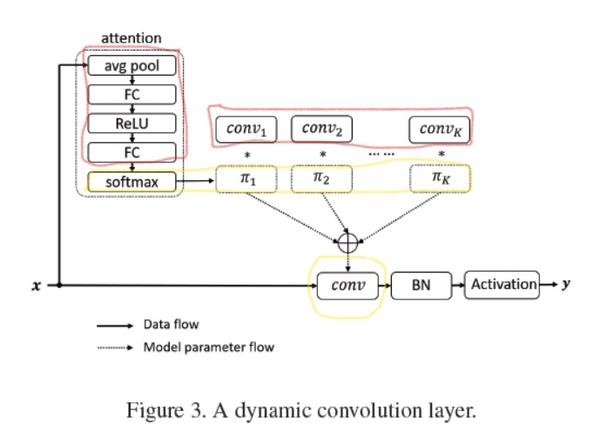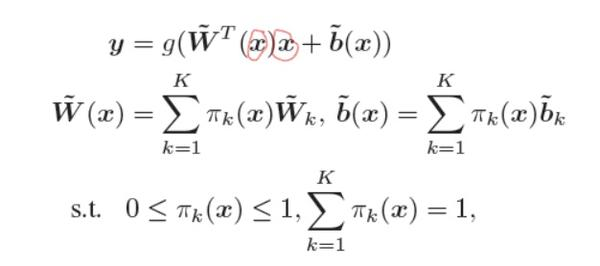# 基于 Pytorch 的动态卷积复现

## 文章简单的回顾class attention2d(nn.Module):
def __init__(self, in_planes, K,):
super(attention2d, self).__init__()
self.fc1 = nn.Conv2d(in_planes, K, 1,)
self.fc2 = nn.Conv2d(K, K, 1,)

def forward(self, x):
x = self.avgpool(x)
x = self.fc1(x)
x = F.relu(x)
x = self.fc2(x).view(x.size(0), -1)
return F.softmax(x, 1)## 分组卷积以及如何通过分组卷积实现 $batch_size$ 大于1的动态卷积

class Dynamic_conv2d(nn.Module):
def __init__(self, in_planes, out_planes, kernel_size, stride=1, padding=0, dilation=1, groups=1, bias=True, K=4,):
super(Dynamic_conv2d, self).__init__()
assert in_planes%groups==0
self.in_planes = in_planes
self.out_planes = out_planes
self.kernel_size = kernel_size
self.stride = stride
self.dilation = dilation
self.groups = groups
self.bias = bias
self.K = K
self.attention = attention2d(in_planes, K, )

self.weight = nn.Parameter(torch.Tensor(K, out_planes, in_planes//groups, kernel_size, kernel_size), requires_grad=True)
if bias:
self.bias = nn.Parameter(torch.Tensor(K, out_planes))
else:
self.bias = None

def forward(self, x):#将batch视作维度变量，进行组卷积，因为组卷积的权重是不同的，动态卷积的权重也是不同的
softmax_attention = self.attention(x)
batch_size, in_planes, height, width = x.size()
x = x.view(1, -1, height, width)# 变化成一个维度进行组卷积
weight = self.weight.view(self.K, -1)

# 动态卷积的权重的生成， 生成的是batch_size个卷积参数（每个参数不同）
aggregate_weight = torch.mm(softmax_attention, weight).view(-1, self.in_planes, self.kernel_size, self.kernel_size)
if self.bias is not None:
aggregate_bias = torch.mm(softmax_attention, self.bias).view(-1)
dilation=self.dilation, groups=self.groups*batch_size)
else:
dilation=self.dilation, groups=self.groups * batch_size)

output = output.view(batch_size, self.out_planes, output.size(-2), output.size(-1))
return output